# class 10 maths solutions## Maths solutions for applications of trigonometry class 10 ssc

Maths solutions for applications of trigonometry class 10 ssc Tenth class mathematics chapter 12 exercises 12.1 and 12.2 solutions are given.  These solutions are very easy to understand. First study the chapter 12 well for many times. Observe the example probems and solutions well. Try them in your own method. Next solve the exercise problems. …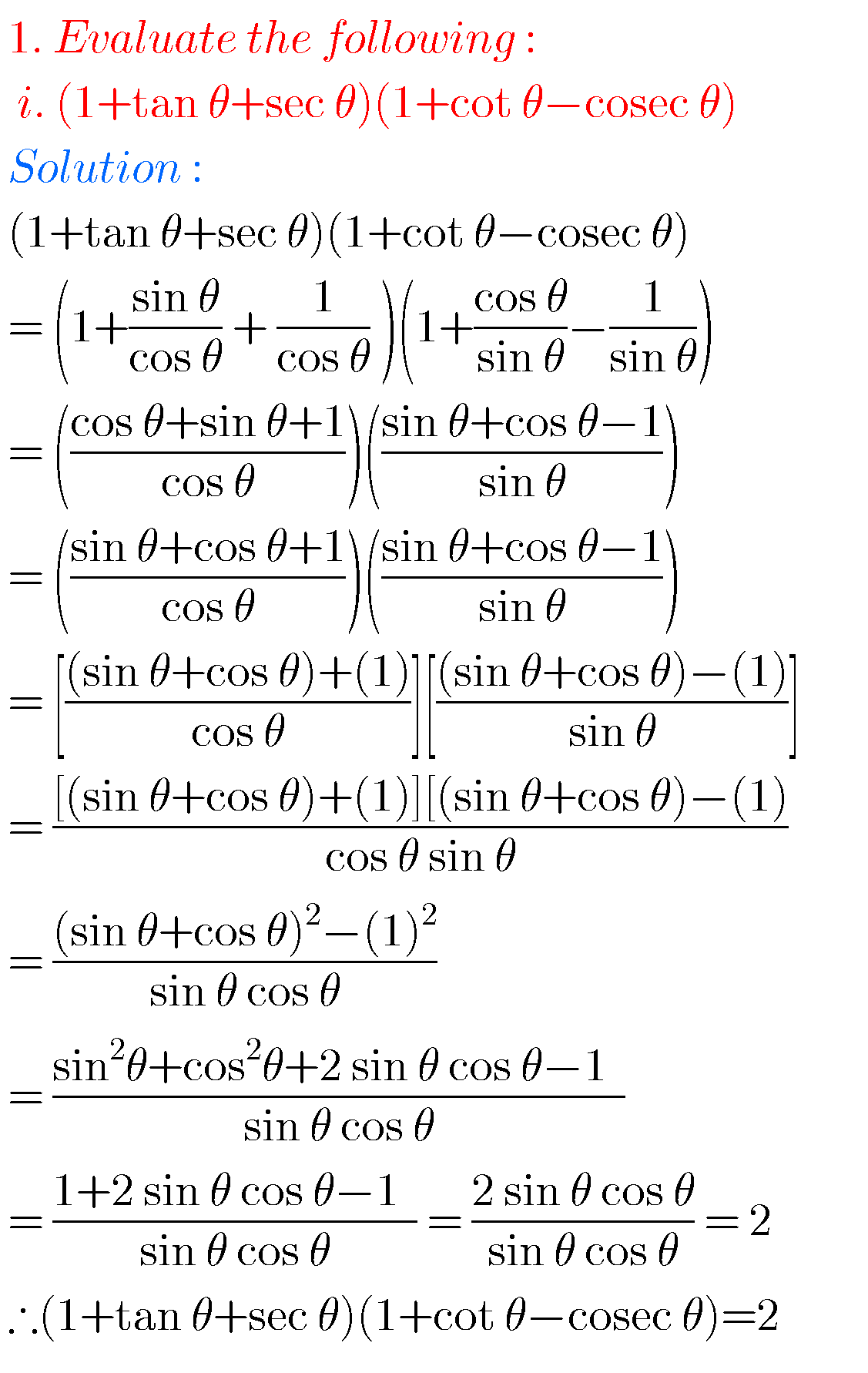## SSC mathematics Trigonometry solutions class 10

Trigonometry solutions class 10 maths ssc Tenth class mathematics chapter 11 trigonometry exercises 11.1, 11.2, 11.3, 11.4, solutions are given. These solutions are very easy to understand. First study the chapter 11 very well. Practice example problems and solutions. Observe the solutions and try them in your own method. Class 10 maths trigonometry solutions You …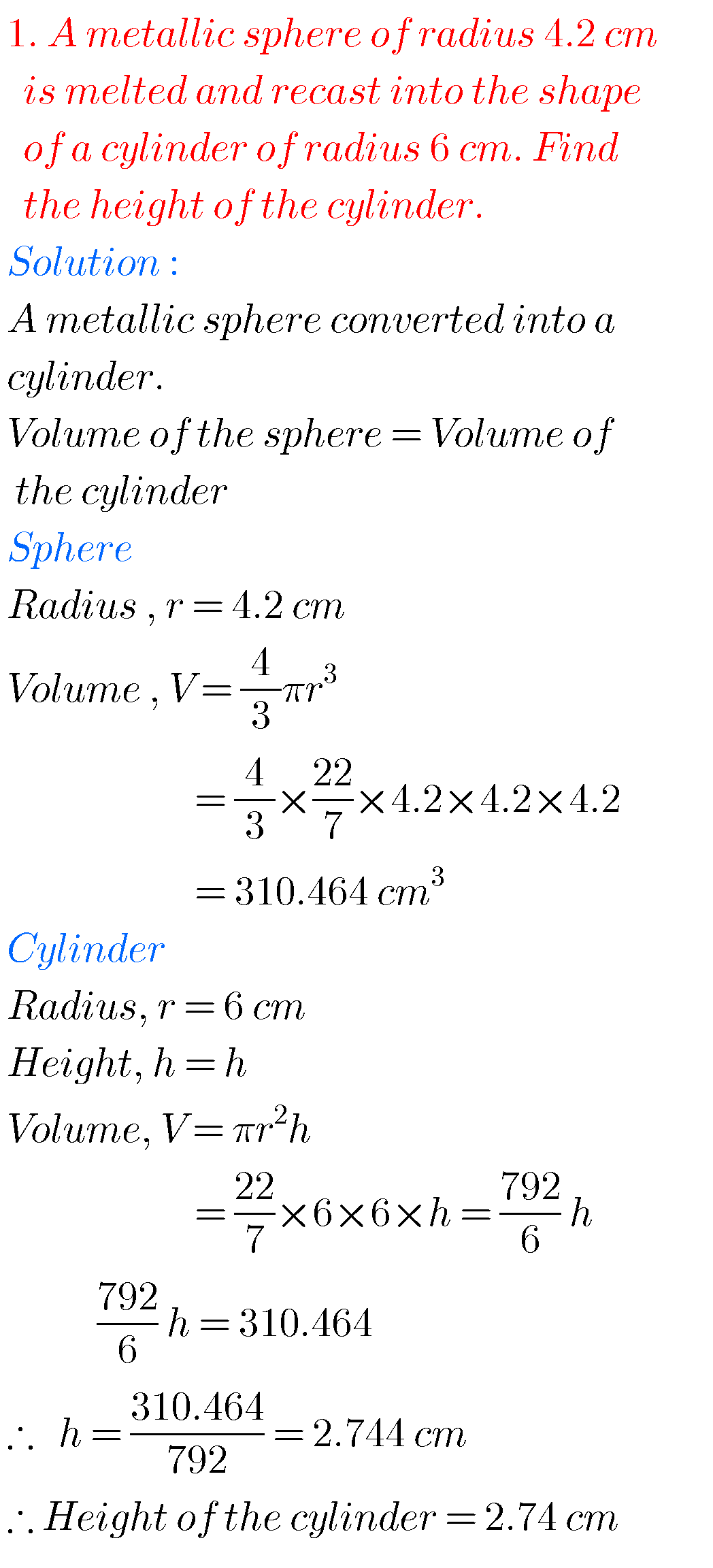## Mensuration solutions class 10 maths ssc

Mensuration solutions class 10 maths ssc SSC maths mensurations solutions for class 10 exercise 10.1, 10.2, 10.3 and 10.4 are given. Study the text book very well. Observe the example problems and solutions. The given solutions are very easy to understand. Observe the solutions and try them in your own method. You can see the …## SSC solutions for maths Progressions class 10

SSC solutions for maths Progressions class 10 Mathematics class 10 SSC Progressions text book problems with solutions are given. These are very easy to understand. First you study the text book very well. Observe the example problems and solutions. Observe the solutions and try them in your own method.  You can also see the solutions …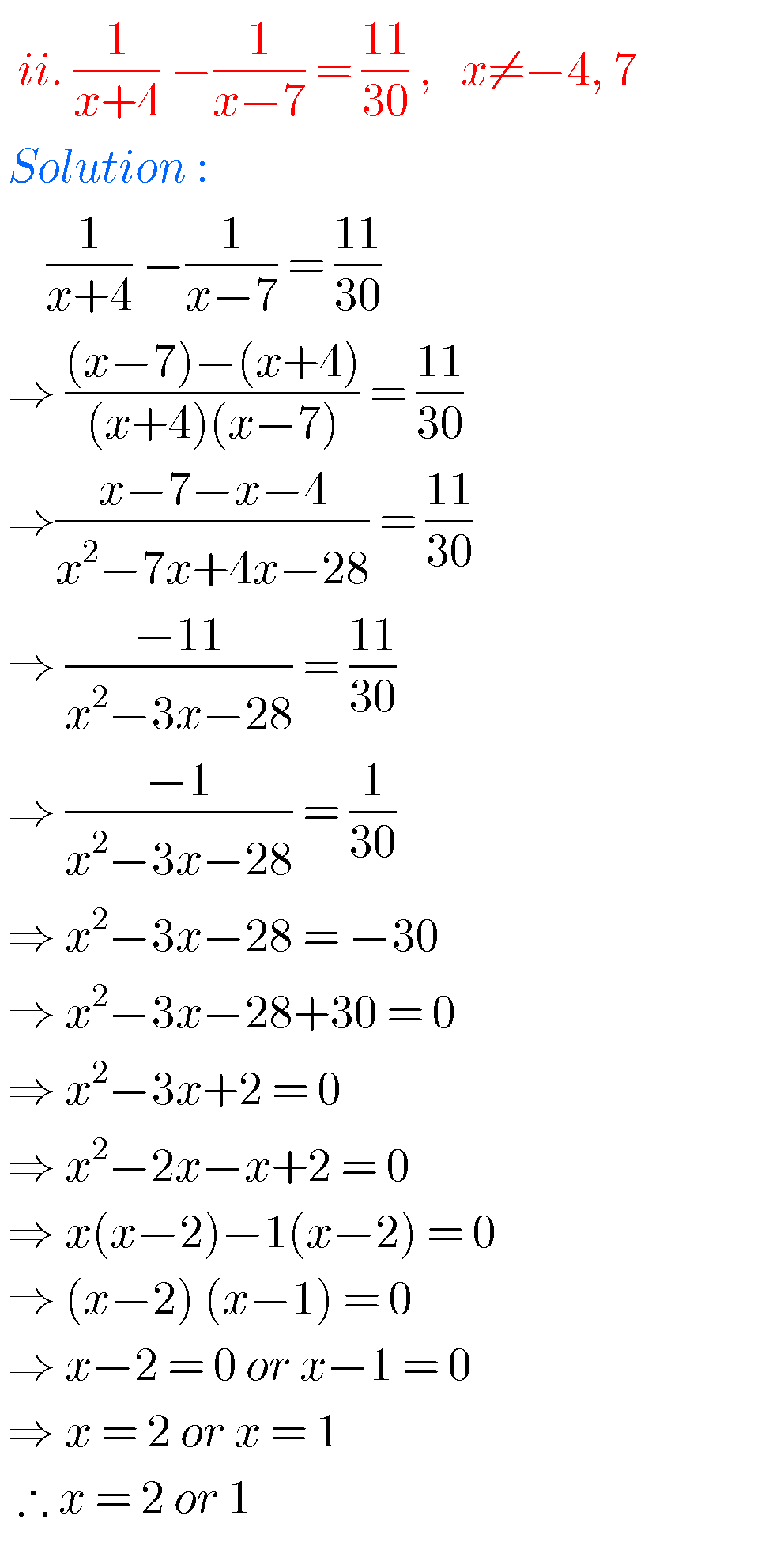## SSC solutions for maths Quadratic equations class 10

SSC solutions for maths Quadratic equations class 10 Mathematics class 10 SSC solutions for Quadratic equations are given. Exercise 5.1, 5.2. 5.3 and 5.4 problems with solutions are given. Observe the solutions and try them in your own method. First you study the text book very well. Observe the example problems and solutions.  You can …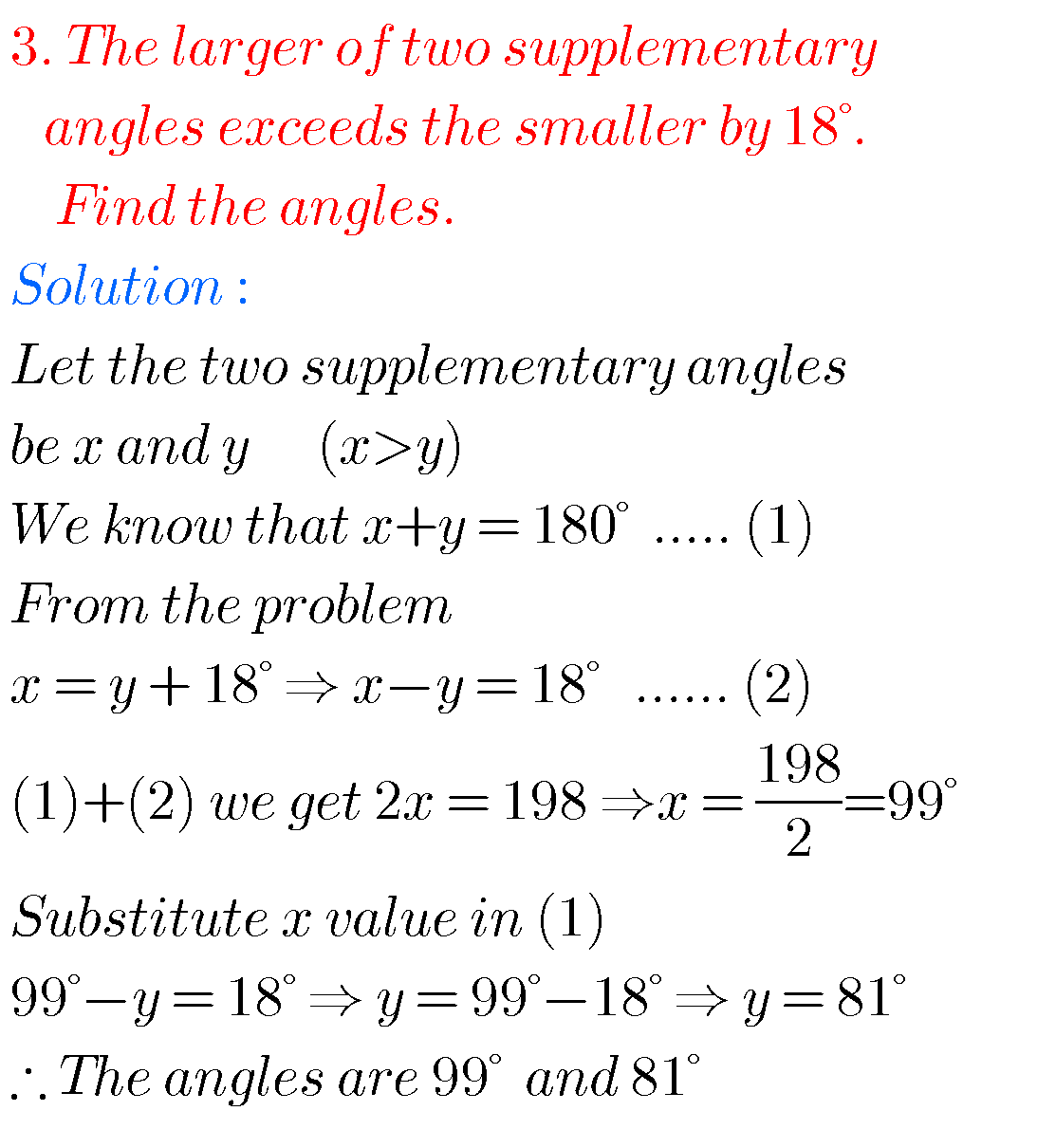## SSC maths solutions for Pair of linear equations in two variables class 10

SSC maths solutions for pair of Linear Equations in Two Variables SSC mathematics class 10 pair of linear equations in two variables solutions for text book exercises. These solutions are very easy to understand. First study the text book well. Exercise 4.1, exercise 4.2 and exercise 4.3  solutions are given.  You can also see the …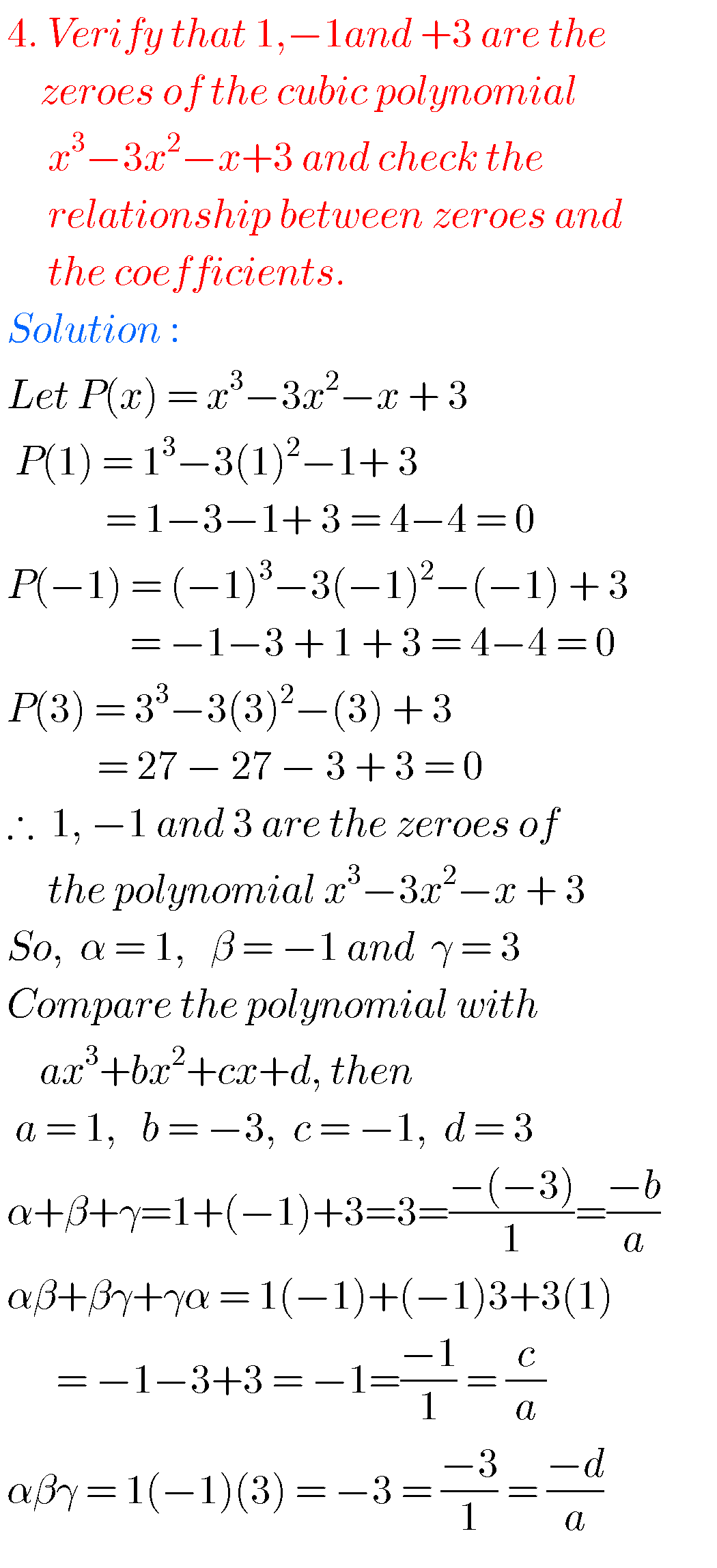## Tenth maths guide for polynomials,exercise 3.1, 3.2, 3.3, 3.4,solutions for class 10 maths chapter 3

Tenth maths guide for polynomials, exercise 3.1, 3.2, 3.3, 3.4. Tenth class mathematics solutions for text book problems. These are very easy to understand. Maths solutions for 10th class Andhra pradesh and Telangana. SSC maths solutions for chapter 3 polynomials. You can also see the solutions for 1. Real numbers 2. Sets 3. Polynomials 4. Pair of linear …## Maths solutions,ssc 10th class real numbers chapter 1,exercise 1.1, 1.2, 1.3, 1.4 and 1.5, ap and ts

Maths solutions for ssc or 10th class real numbers chapter 1 (ap and ts ) You can see the solutions for maths 10th class real numbers for some problems ( text book ). These solutions are very easy to understand. Andhra pradesh and telangana 10 th class mathematics solutions for real numbers. SSC maths solutions …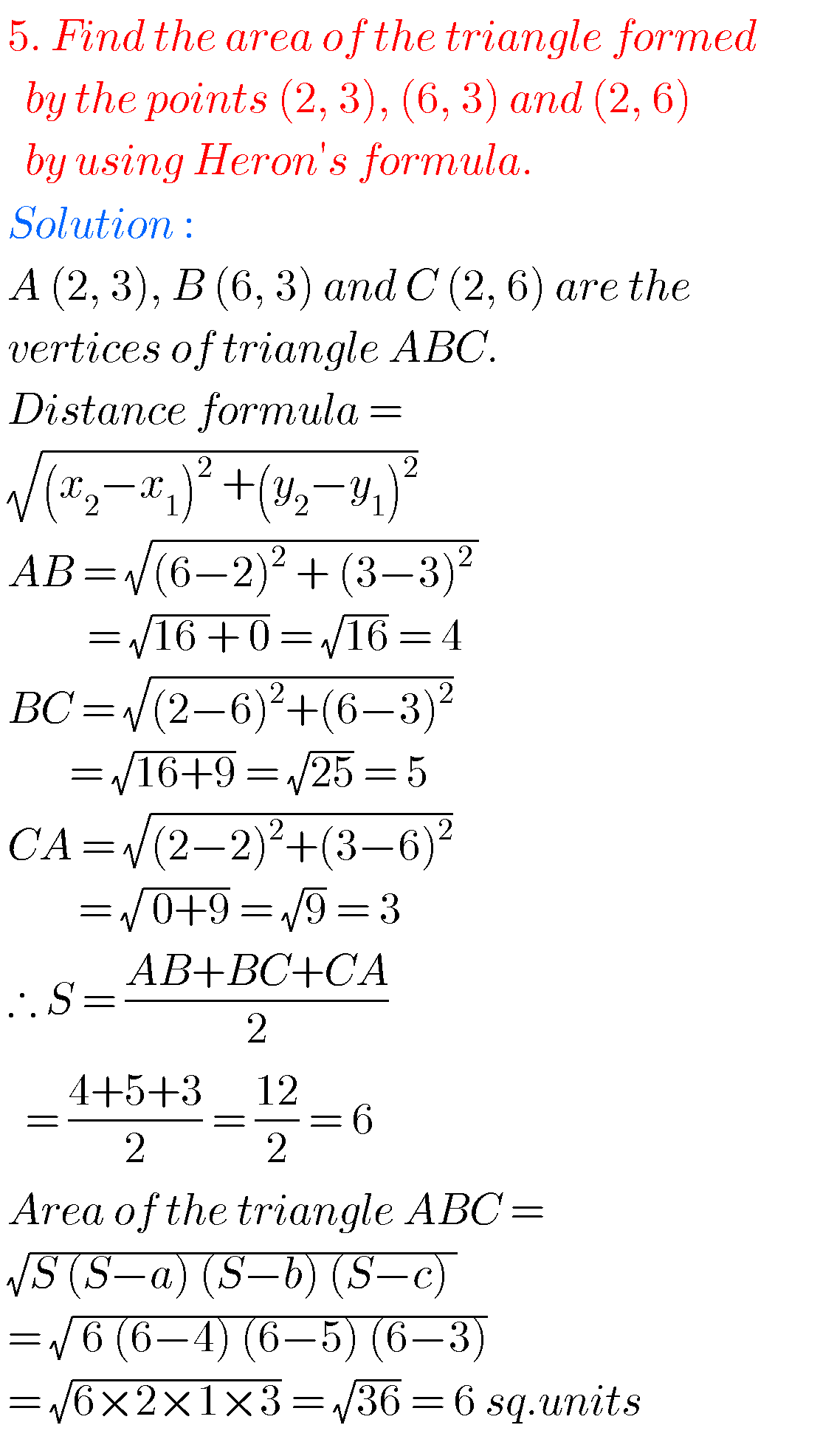## SSC solutions for maths Coordinate Geometry class 10, AP & TS

SSC solutions for maths Coordinate Geometry Mathematics solutions for Coordinate Geometry class 10 SSC. Exercise 7.1, exercise 7.2, exercise 7.3, and exercise 7.4 solutions are given. Thsese solutions are very easy to understand. First study the text book very well. Observe the example problems and solutions. Observe the solutions and try them in your own …